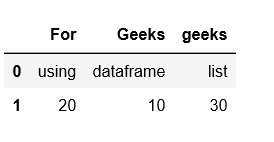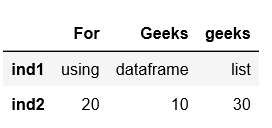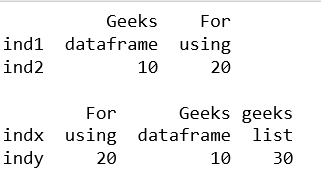Related Articles

# Create a Pandas DataFrame from List of Dicts

• Last Updated : 17 Dec, 2018

Pandas DataFrame is a 2-dimensional labeled data structure with columns of potentially different types. It is generally the most commonly used pandas object.

Pandas DataFrame can be created in multiple ways. Let’s discuss how to create a Pandas DataFrame from List of Dicts.

Attention geek! Strengthen your foundations with the Python Programming Foundation Course and learn the basics.

To begin with, your interview preparations Enhance your Data Structures concepts with the Python DS Course. And to begin with your Machine Learning Journey, join the Machine Learning - Basic Level Course

Code #1:

 `# Python code demonstrate how to create  ``# Pandas DataFrame by lists of dicts. ``import` `pandas as pd ``   ` `# Initialise data to lists. ``data ``=` `[{``'Geeks'``: ``'dataframe'``, ``'For'``: ``'using'``, ``'geeks'``: ``'list'``},``        ``{``'Geeks'``:``10``, ``'For'``: ``20``, ``'geeks'``: ``30``}] ``   ` `# Creates DataFrame. ``df ``=` `pd.DataFrame(data) ``   ` `# Print the data ``df `

Output:Code #2: With `index`

 `# Python code demonstrate how to create  ``# Pandas DataFrame by lists of dicts. ``import` `pandas as pd ``   ` `# Initialise data to lists. ``data ``=` `[{``'Geeks'``: ``'dataframe'``, ``'For'``: ``'using'``, ``'geeks'``: ``'list'``},``        ``{``'Geeks'``:``10``, ``'For'``: ``20``, ``'geeks'``: ``30``}] ``   ` `# Creates DataFrame. ``df ``=` `pd.DataFrame(data, index ``=``[``'ind1'``, ``'ind2'``]) ``   ` `# Print the data ``df `

Output:Code #3: With `index` and `columns`

 `# Python code demonstrate how to create  ``# Pandas DataFrame by lists of dicts. ``import` `pandas as pd ``   ` `# Initialise data to lists. ``data ``=` `[{``'Geeks'``: ``'dataframe'``, ``'For'``: ``'using'``, ``'geeks'``: ``'list'``},``        ``{``'Geeks'``:``10``, ``'For'``: ``20``, ``'geeks'``: ``30``}] ``   ` `# With two column indices, values same  ``# as dictionary keys ``df1 ``=` `pd.DataFrame(data, index ``=``[``'ind1'``, ``'ind2'``],``                      ``columns ``=``[``'Geeks'``, ``'For'``]) ``    ` `# With two column indices with  ``# one index with other name ``df2 ``=` `pd.DataFrame(data, index ``=``[``'indx'``, ``'indy'``]) ``    ` `# print for first data frame ``print` `(df1, ``"\n"``) ``    ` `# Print for second DataFrame. ``print` `(df2) `

Output:My Personal Notes arrow_drop_up